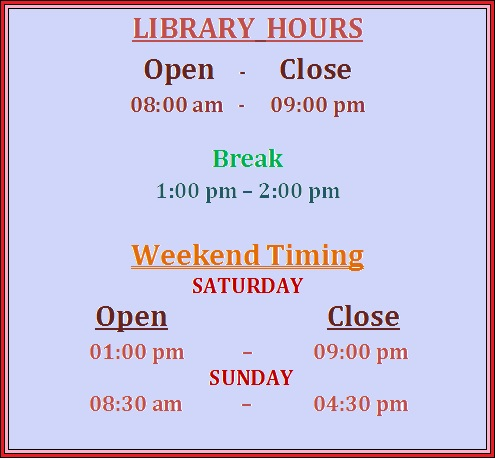# Introduction to Operations Research: Home

To understand different application areas of operations research like transportation problem, assignment model, sequencing models, dynamic programming, game theory, replacement models & inventory models.

## Objectives

To understand definition, scope, objectives, phases, models & limitations of operations research. To understand different application areas of operations research like transportation problem, assignment model, sequencing models, dynamic programming, game theory, replacement models & inventory models. Formulate simple reasoning, learning and optimization problems, in terms of the representations and methods presented. Manipulate the basic mathematical structures underlying these methods, such as system state, search trees, plan spaces, model theory, propositional logic, constraint systems, Markov decision processes, decision trees, linear programs and integer programs. Demonstrate the hand execution of basic reasoning and optimization algorithms on simple problems. Formulate more complex, but still relatively simple problems, and apply implementations of selected algorithms to solve these problems. Evaluate analytically the limitations of these algorithms, and assess tradeoffs between these algorithms.

## Level## Library Timing## Search the Library Catalog

Welcome to the Online Public Access Catalog (OPAC). This catalog provides you bibliographic information of print books available in all campus libraries of the University and provides you the facility of 2 types of searches; basic search and advance search to find the exact book or list of books you required.